# Building A Neural Net from Scratch Using R - Part 1

by Akshaj Verma

Akshaj is a budding deep learning researcher who loves to work with R. He has worked as a Research Associate at the Indian Institute of Science and as a Data Scientist at KPMG India.

A lot of deep learning frameworks often abstract away the mechanics behind training a neural network. While this has the advantage of quickly building deep learning models, it has the disadvantage of hiding the details. It is equally important to slow down and understand how neural nets work. In this two-part series, we’ll dig deep and build our own neural net from scratch. This will help us understand, at a basic level, how those big frameworks work. The network we’ll build will contain a single hidden layer and perform binary classification using a vectorized implementation of backpropagation, all written in base-R. We will describe in detail what a single-layer neural network is, how it works, and the equations used to describe it. We will see what kind of data preparation is required to be able to use it with a neural network. Then, we will implement a neural-net step-by-step from scratch and examine the output at each step. Finally, to see how our neural-net fares, we will describe a few metrics used for classification problems and use them.

In this first part, we’ll present the dataset we are going to use, the pre-processing involved, the train-test split, and describe in detail the architecture of the model. Then we’ll build our neural net chunk-by-chunk. It will involve writing functions for initializing parameters and running forward propagation.

In the second part, we’ll implement backpropagation by writing functions to calculate gradients and update the weights. Finally, we’ll make predictions on the test data and see how accurate our model is using metrics such as Accuracy, Recall, Precision, and F1-score. We’ll compare our neural net with a logistic regression model and visualize the difference in the decision boundaries produced by these models.

By the end of this series, you should have a deeper understanding of the math behind neural-networks and the ability to implement it yourself from scratch!

### Set Seed

Before we start, let’s set a seed value to ensure reproducibility of the results.

set.seed(69)

### Architecture Definition

To understand the matrix multiplications better and keep the numbers digestible, we will describe a very simple 3-layer neural net i.e. a neural net with a single hidden layer. The $$1^{st}$$ layer will take in the inputs and the $$3^{rd}$$ layer will spit out an output.

The input layer will have two (input) neurons, the hidden layer four (hidden) neurons, and the output layer one (output) neuron.

Our input layer has two neurons because we’ll be passing two features (columns of a dataframe) as the input. A single output neuron because we’re performing binary classification. This means two output classes - 0 and 1. Our output will actually be a probability (a number that lies between 0 and 1). We’ll define a threshold for rounding off this probability to 0 or 1. For instance, this threshold can be 0.5.

In a deep neural net, multiple hidden layers are stacked together (hence the name “deep”). Each hidden layer can contain any number of neurons you want.

In this series, we’re implementing a single-layer neural net which, as the name suggests, contains a single hidden layer.

• n_x: the size of the input layer (set this to 2).
• n_h: the size of the hidden layer (set this to 4).
• n_y: the size of the output layer (set this to 1).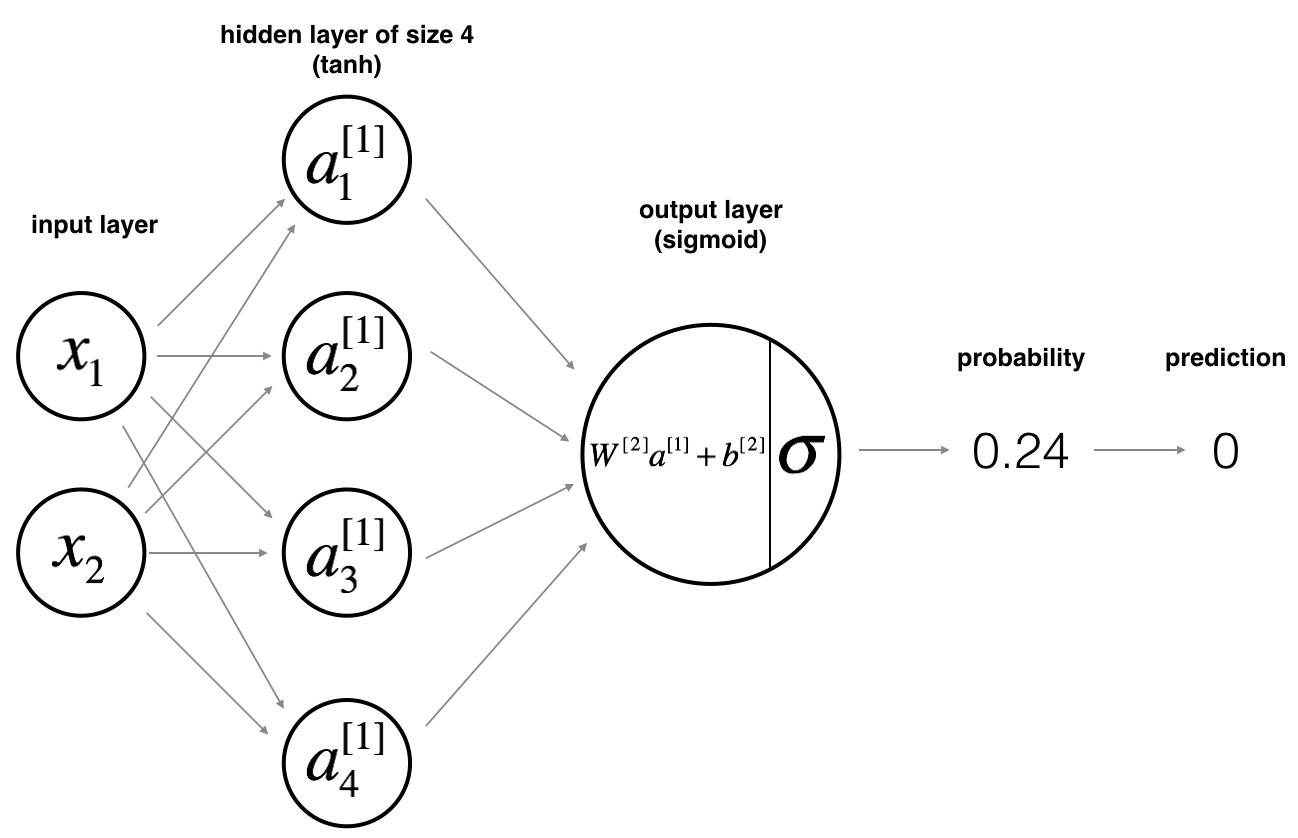Neural networks flow from left to right, i.e. input to output. In the above example, we have two features (two columns from the input dataframe) that arrive at the input neurons from the first-row of the input dataframe. These two numbers are then multiplied by a set of weights (randomly initialized at first and later optimized).

An activation function is then applied on the result of this multiplication. This new set of numbers becomes the neurons in our hidden layer. These neurons are again multiplied by another set of weights (randomly initialized) with an activation function applied to this result. The final result we obtain is a single number. This is the prediction of our neural-net. It’s a number that lies between 0 and 1.

Once we have a prediction, we then compare it to the true output. To optimize the weights in order to make our predictions more accurate (because right now our input is being multiplied by random weights to give a random prediction), we need to first calculate how far off is our prediction from the actual value. Once we have this loss, we calculate the gradients with respect to each weight.

The gradients tell us the amount by which we need to increase or decrease each weight parameter in order to minimize the loss. All the weights in the network are updated as we repeat the entire process with the second input sample (second row).

After all the input samples have been used to optimize weights, we say that one epoch has passed. We repeat this process for multiple number of epochs till our loss stops decreasing.

At this point, you might be wondering what an activation function is. An activation function adds non-linearity to our network and enables it to learn complex features. If you look closely, a neural network consists of a bunch multiplications and additions. It’s linear and we know that a linear classification model will not be able to learn complex features in high dimensions.

Here are a few popular activation functions -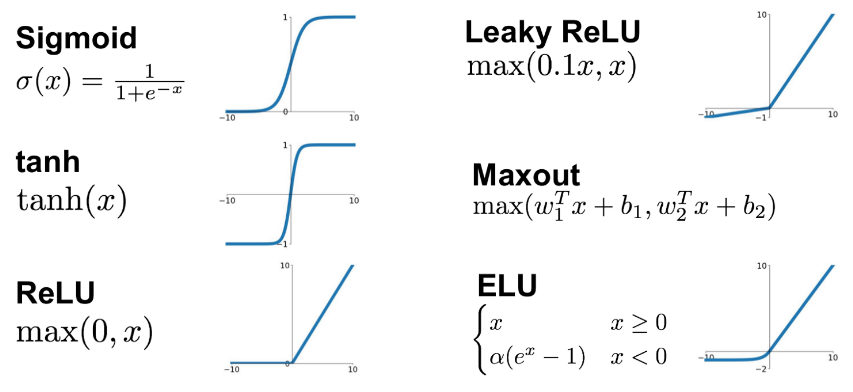We will use tanh() and sigmoid() activation functions in our neural net. Because tanh() is already available in base-R, we will implement the sigmoid() function ourselves later on.

### Dry Run

For now, let’s see how the numbers flow through the above described neural-net by writing out the equations for a single sample (one input row).

For one input sample $$x^{(i)}$$ where $$i$$ is the row-number:

First, we calculate the output $$Z$$ from the input $$x$$. We will tune the parameters $$W$$ and $$b$$. Here, the superscript in square brackets tell us the layer number and the one in parenthesis tell use the neuron number. For instance $$z^{ (i)}$$ is the output from the $$i^{{th}}$$ neuron of the $$1^{{st}}$$ layer.

$z^{ (i)} = W^{} x^{(i)} + b^{ (i)}\tag{1}$

Then we’ll pass this value through the tanh() activation function to get $$a$$.

$a^{ (i)} = \tanh(z^{ (i)})\tag{2}$

After that, we’ll calculate the value for the final output layer using the hidden layer values.

$z^{ (i)} = W^{} a^{ (i)} + b^{ (i)}\tag{3}$

Finally, we’ll pass this value through the sigmoid() activation function and obtain our output probability. $\hat{y}^{(i)} = a^{ (i)} = \sigma(z^{  (i)})\tag{4}$

To obtain our prediction class from output probabilities, we round off the values as follows. $y^{(i)}_{prediction} = \begin{cases} 1 & \mbox{if } a^{(i)} > 0.5 \\ 0 & \mbox{otherwise } \end{cases}\tag{5}$

Once, we have the prediction probabilities, we’ll compute the loss in order to tune our parameters ($$w$$ and $$b$$ can be adjusted using gradient-descent).

Given the predictions on all the examples, we will compute the cost $$J$$ the cross-entropy loss as follows:
$J = - \frac{1}{m} \sum\limits_{i = 0}^{m} \large\left(\small y^{(i)}\log\left(\hat{y}^{(i)}\right) + (1-y^{(i)})\log\left(1- \hat{y}^{ (i)}\right) \large \right) \small \tag{6}$

Once we have our loss, we need to calculate the gradients. I’ve calculated them for you so you don’t differentiate anything. We’ll directly use these values -

• $$dZ^{} = A^{} - Y$$
• $$dW^{} = \frac{1}{m} dZ^{}A^{^T}$$
• $$db^{} = \frac{1}{m}\sum dZ^{}$$
• $$dZ^{} = W^{^T} * g^{'} Z^{}$$ where $$g$$ is the activation function.
• $$dW^{} = \frac{1}{m}dZ^{}X^{T}$$
• $$db^{} = \frac{1}{m}\sum dZ^{}$$

Now that we have the gradients, we will update the weights. We’ll multiply these gradients with a number known as the learning rate. The learning rate is represented by $$\alpha$$.

• $$W^{} = W^{} - \alpha * dW^{}$$
• $$b^{} = b^{} - \alpha * db^{}$$
• $$W^{} = W^{} - \alpha * dW^{}$$
• $$b^{} = b^{} - \alpha * db^{}$$

This process is repeated multiple times until our model converges i.e. we have learned a good set of weights that fit our data well.

## Load and Visualize the Data

Since, the goal of the series is to understand how neural-networks work behind the scene, we’ll use a small dataset so that our focus is on building our neural net.

We’ll use a planar dataset that looks like a flower. The output classes cannot be separated accurately using a straight line.

### Construct Dataset

df <- read.csv(file = "planar_flower.csv")

Let’s shuffle our dataset so that our model is invariant to the order of samples. This is good for generalization and will help increase performance on unseen (test) data.

df <- df[sample(nrow(df)), ]

head(df)
##           x1        x2 y
## 209  1.53856  3.242555 0
## 347 -0.05617 -0.808464 0
## 386 -3.85811  1.423514 1
## 112  0.82630  0.044276 1
## 104  0.31350  0.004274 1
## 111  2.28420  0.352476 1

### Visualize Data

We have four hundred samples where two hundred belong each class.

Here’s a scatter plot between our input variables. As you can see, the output classes are not easily separable.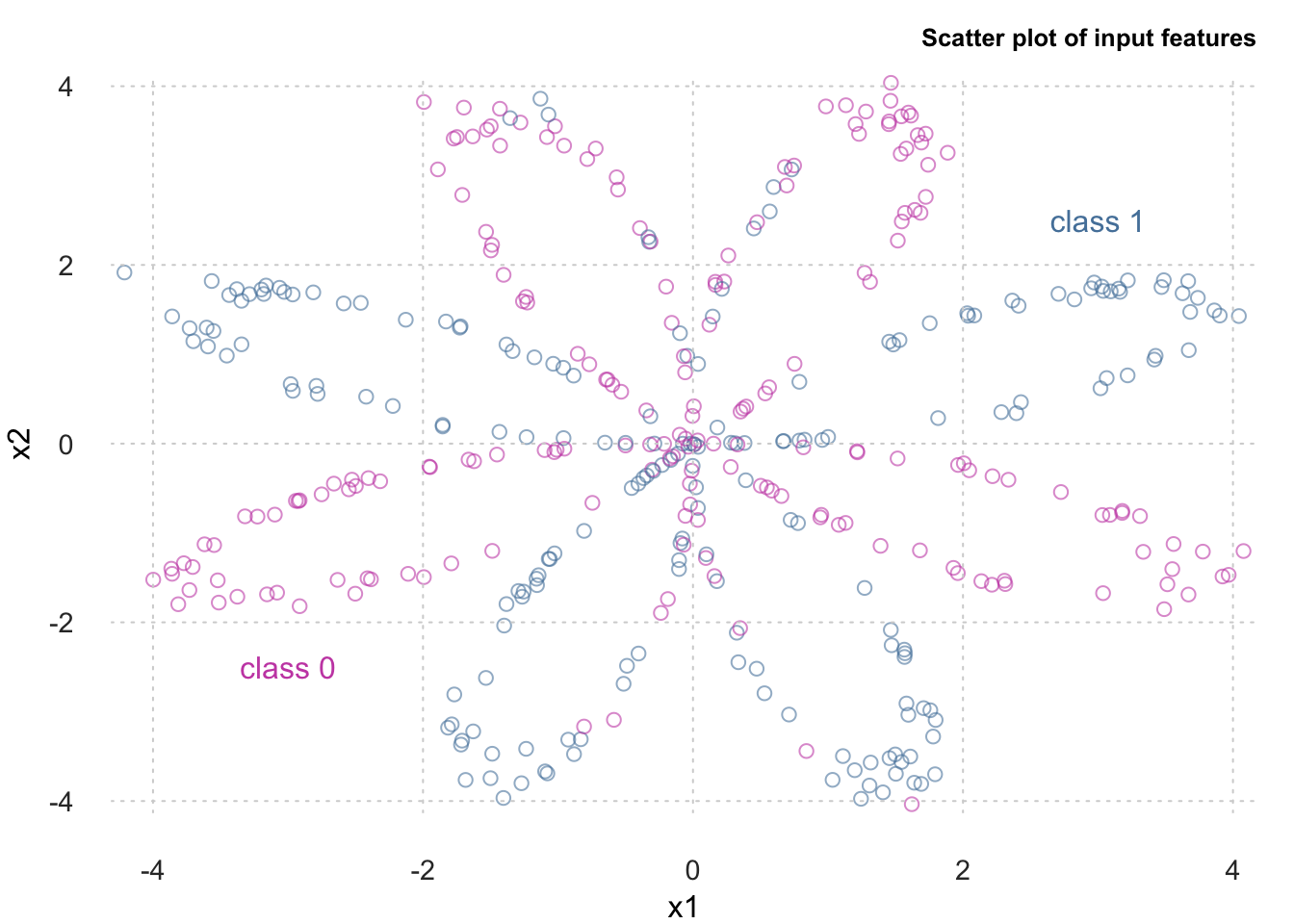### Train-Test Split

Now that we have our dataset prepared, let’s go ahead and split it into train and test sets. We’ll put 80% of our data into our train set and the remaining 20% into our test set. (To keep the focus on the neural-net, we will not be using a validation set here. ).

train_test_split_index <- 0.8 * nrow(df)

#### Train and Test Dataset

Because we’ve already shuffled the dataset above, we can go ahead and extract the first 80% rows into train set.

train <- df[1:train_test_split_index,]
head(train)
##           x1        x2 y
## 209  1.53856  3.242555 0
## 347 -0.05617 -0.808464 0
## 386 -3.85811  1.423514 1
## 112  0.82630  0.044276 1
## 104  0.31350  0.004274 1
## 111  2.28420  0.352476 1

Next, we select last 20% rows of the shuffled dataset to be our test set.

test <- df[(train_test_split_index+1): nrow(df),]
head(test)
##          x1       x2 y
## 210 -0.0352 -0.03489 0
## 348  2.7257 -0.54170 0
## 19  -2.2235  0.42137 1
## 362  2.3366 -0.40412 0
## 143 -1.4984  3.55267 0
## 4   -3.2264 -0.81648 0

Here, we visualize the number of samples per class in our train and test data sets to ensure that there isn’t a major class imbalance.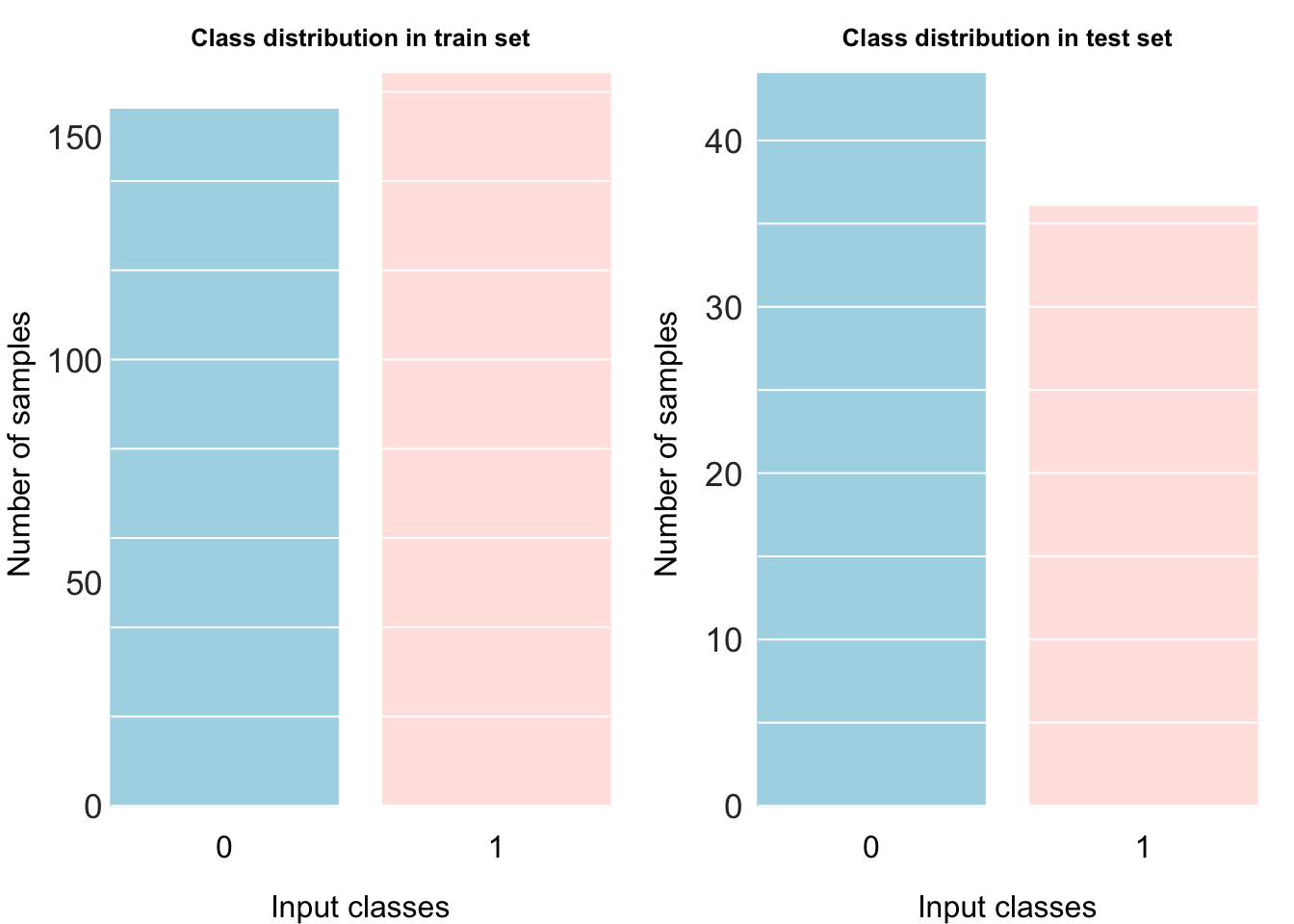## Preprocess

Neural networks work best when the input values are standardized. So, we’ll scale all the values to to have their mean=0 and standard-deviation=1.

Standardizing input values speeds up the training and ensures faster convergence.

To standardize the input values, we’ll use the scale() function in R. Note that we’re standardizing the input values (X) only and not the output values (y).

X_train <- scale(train[, c(1:2)])

y_train <- train$y dim(y_train) <- c(length(y_train), 1) # add extra dimension to vector X_test <- scale(test[, c(1:2)]) y_test <- test$y
dim(y_test) <- c(length(y_test), 1) # add extra dimension to vector

The output below tells us the shape and size of our input data.

## Shape of X_train (row, column):
##  320 2
## Shape of y_train (row, column) :
##  320 1
## Number of training samples:
##  320
## Shape of X_test (row, column):
##  80 2
## Shape of y_test (row, column) :
##  80 1
## Number of testing samples:
##  80

Because neural nets are made up of a bunch matrix multiplications, let’s convert our input and output to matrices from dataframes. While dataframes are a good way to represent data in a tabular form, we choose to convert to a matrix type because matrices are smaller than an equivalent dataframe and often speed up the computations.

We will also change the shape of X and y by taking its transpose. This will make the matrix calculations slightly more intuitive as we’ll see in the second part. There’s really no difference though. Some of you might find this way better, while others might prefer the non-transposed way. I feel this this makes more sense.

We’re going to use the as.matrix() method to construct out matrix. We’ll fill out matrix row-by-row.

X_train <- as.matrix(X_train, byrow=TRUE)
X_train <- t(X_train)
y_train <- as.matrix(y_train, byrow=TRUE)
y_train <- t(y_train)

X_test <- as.matrix(X_test, byrow=TRUE)
X_test <- t(X_test)
y_test <- as.matrix(y_test, byrow=TRUE)
y_test <- t(y_test)

Here are the shapes of our matrices after taking the transpose.

## Shape of X_train:
##  2 320
## Shape of y_train:
##  1 320
## Shape of X_test:
##  2 80
## Shape of y_test:
##  1 80

## Build a neural-net

Now that we’re done processing our data, let’s move on to building our neural net. As discussed above, we will broadly follow the steps outlined below.

1. Define the neural net architecture.
2. Initialize the model’s parameters from a random-uniform distribution.
3. Loop:
• Implement forward propagation.
• Compute loss.
• Implement backward propagation to get the gradients.
• Update parameters.

### Get layer sizes

A neural network optimizes certain parameters to get to the right output. These parameters are initialized randomly. However, the size of these matrices is dependent upon the number of layers in different layers of neural-net.

To generate matrices with random parameters, we need to first obtain the size (number of neurons) of all the layers in our neural-net. We’ll write a function to do that. Let’s denote n_x, n_h, and n_y as the number of neurons in input layer, hidden layer, and output layer respectively.

We will obtain these shapes from our input and output data matrices created above.

dim(X) gives us $$2$$ because the shape of X is (2, 320). We do the same for dim(y).

getLayerSize <- function(X, y, hidden_neurons, train=TRUE) {
n_x <- dim(X)
n_h <- hidden_neurons
n_y <- dim(y)

size <- list("n_x" = n_x,
"n_h" = n_h,
"n_y" = n_y)

return(size)
}

As we can see below, the number of neurons is decided based on shape of the input and output matrices.

layer_size <- getLayerSize(X_train, y_train, hidden_neurons = 4)
layer_size
## $n_x ##  2 ## ##$n_h
##  4
##
## $n_y ##  1 ### Initialise parameters Before we start training our parameters, we need to initialize them. Let’s initialize the parameters based on random uniform distribution. The function initializeParameters() takes as argument an input matrix and a list which contains the layer sizes i.e. number of neurons. The function returns the trainable parameters W1, b1, W2, b2. Our neural-net has 3 layers, which gives us 2 sets of parameter. The first set is W1 and b1. The second set is W2 and b2. Note that these parameters exist as matrices. These random weights matrices W1, b1, W2, b2 are created based on the layer sizes of the different layers (n_x, n_h, and n_y). The sizes of these weights matrices are - W1 = (n_h, n_x) b1 = (n_h, 1) W2 = (n_y, n_h) b2 = (n_y, 1) initializeParameters <- function(X, list_layer_size){ m <- dim(data.matrix(X)) n_x <- list_layer_size$n_x
n_h <- list_layer_size$n_h n_y <- list_layer_size$n_y

W1 <- matrix(runif(n_h * n_x), nrow = n_h, ncol = n_x, byrow = TRUE) * 0.01
b1 <- matrix(rep(0, n_h), nrow = n_h)
W2 <- matrix(runif(n_y * n_h), nrow = n_y, ncol = n_h, byrow = TRUE) * 0.01
b2 <- matrix(rep(0, n_y), nrow = n_y)

params <- list("W1" = W1,
"b1" = b1,
"W2" = W2,
"b2" = b2)

return (params)
}

For our network, the size of our weight matrices are as follows. Remember that, number of input neurons n_x = 2, hidden neurons n_h = 4, and output neuron n_y = 1. layer_size is calculate above.

init_params <- initializeParameters(X_train, layer_size)
lapply(init_params, function(x) dim(x))
## $W1 ##  4 2 ## ##$b1
##  4 1
##
## $W2 ##  1 4 ## ##$b2
##  1 1

### Define the Activation Functions.

We implement the sigmoid() activation function for the output layer.

sigmoid <- function(x){
return(1 / (1 + exp(-x)))
}

$S(x) = \frac {1} {1 + e^{-x}}$

The tanh() function is already present in R.

$T(x) = \frac {e^x - e^{-x}} {e^x + e^{-x}}$

Here, we plot both activation functions side-by-side for comparison.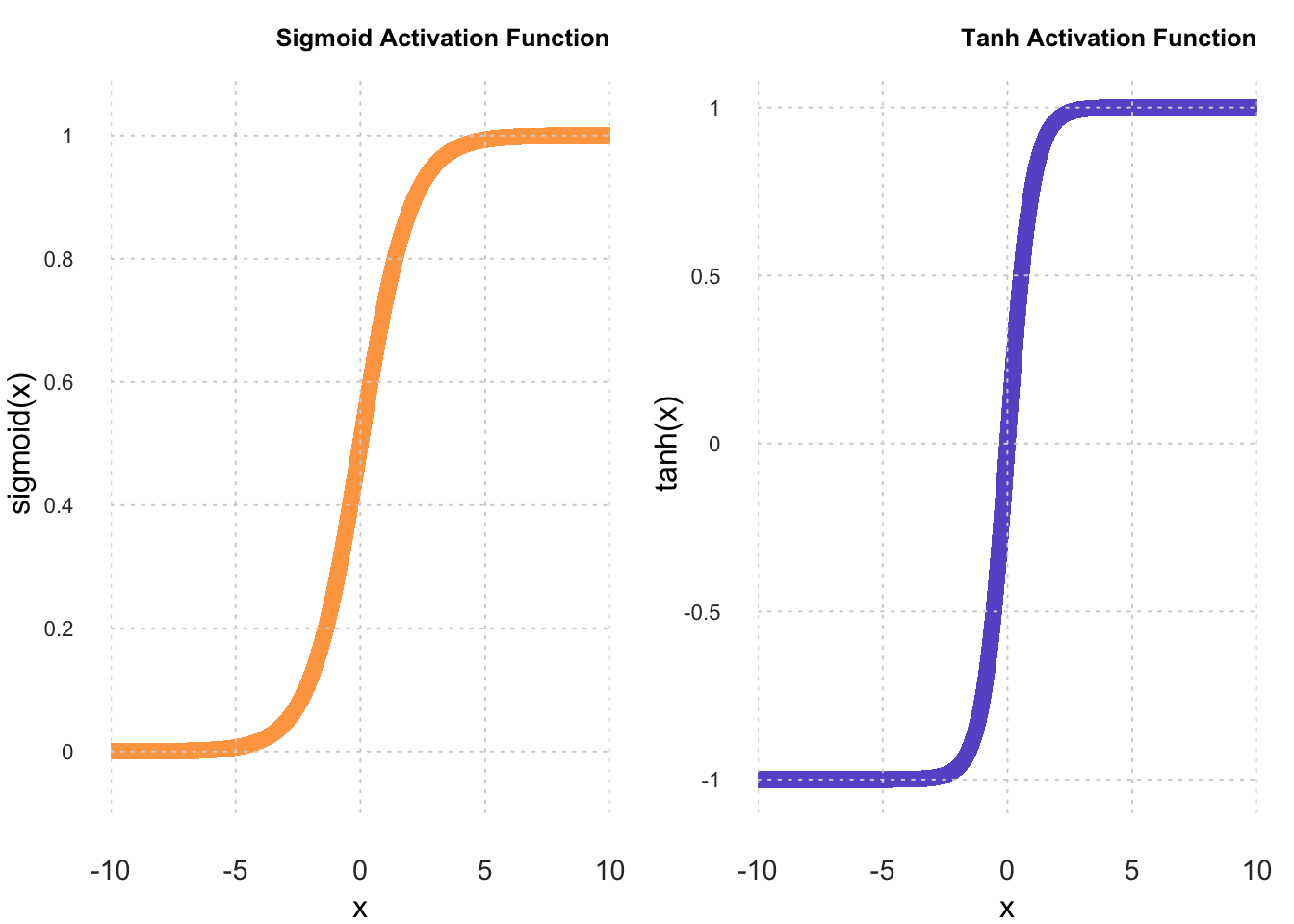### Forward Propagation

Now, onto defining the forward propagation. The function forwardPropagation() takes as arguments the input matrix X, the parameters list params, and the list of layer_sizes. We extract the layers sizes and weights from the respective functions defined above. To perform matrix multiplication, we use the %*% operator.

Before we perform the matrix multiplications, we need to reshape the parameters b1 and b2. Why do we do this? Let’s find out. Note that, the parameter shapes are:

• W1: (4, 2)
• b1: (4, 1)
• W2: (1, 4)
• b2 : (1, 1)

And the layers sizes are:

• n_x = 2
• n_h = 4
• n_y = 1

Finally, shape of input matrix $$X$$ (input layer):

• X: (2, 320)

If we talk about the input => hidden; the hidden layer obtained by the equation A1 = activation(y1) = W1 %*% X + b1, would be as follows:

• For the matrix multiplication of W1 and X, their shapes are correct by default: (4, 2) x (2, 320). The shape of the output matrix W1 %*% X is (4, 320).

• Now, b1 is of shape (4, 1). Since, W1 %*% X is of the shape (4, 320), we need to repeat it b1 320 times, one for each input sample We do that using the command rep(b1, m) where m is calculated as dim(X) which selects the second dimension of the shape of X.

• The shape of A1 is (4, 320).

In the case of hidden => output; the output obtained by the equation y2 = W2 %*% A1 + b2, would be as follows:

• To shapes of W2 and A1 are correct for us to perform matrix multiplication on them. W2 is (1, 4) and A1 is (4, 320). The output W2 %*% A1 has the shape (1, 320). b2 has a shape of (1, 1). We will again repeat b2 like we did above. So, b2 now becomes (1, 320).

• The shape of A2 is now (1, 320).

We use the tanh() activation for the hidden layer and sigmoid() activation for the output layer.

forwardPropagation <- function(X, params, list_layer_size){

m <- dim(X)
n_h <- list_layer_size$n_h n_y <- list_layer_size$n_y

W1 <- params$W1 b1 <- params$b1
W2 <- params$W2 b2 <- params$b2

b1_new <- matrix(rep(b1, m), nrow = n_h)
b2_new <- matrix(rep(b2, m), nrow = n_y)

Z1 <- W1 %*% X + b1_new
A1 <- sigmoid(Z1)
Z2 <- W2 %*% A1 + b2_new
A2 <- sigmoid(Z2)

cache <- list("Z1" = Z1,
"A1" = A1,
"Z2" = Z2,
"A2" = A2)

return (cache)
}

Even though we only need the value A2 for forward propagation, you’ll notice we return all other calculated values as well. We do this because these values will be needed during backpropagation. Saving them here will reduce the the time it takes for backpropagation because we don’t have to calculate it again.

Another thing to notice is the Z and A of a particular layer will always have the same shape. This is because A = activation(Z) which does not change the shape of Z. An activation function only introduces non-linearity in a network.

fwd_prop <- forwardPropagation(X_train, init_params, layer_size)
lapply(fwd_prop, function(x) dim(x))
## $Z1 ##  4 320 ## ##$A1
##    4 320
##
## $Z2 ##  1 320 ## ##$A2
##    1 320

## End of Part 1

We have reached the end of Part1 here. In the next and final part, we will implement backpropagation and evaluate our model. Stay tuned!